Journal of Multimedia Information System
Korea Multimedia Society
Section A

# New Approach to Two-wheeler Detection using Correlation Coefficient based on Histogram of Oriented Gradients

Yeunghak Lee1,*, Jaechang Shim2
1Department of Avionics Engineering College of Aviation, Kyungwoon University, Republic of Korea, annaturu@ikw.ac.kr
2Department of Computer Engineering, College of Engineering, Andong National University, Republic of Korea, jcshim@andong.ac.kr
*Corresponding Author: Yeunghak Lee, Department of Avionics Engineering College of Aviation, Kyungwoon University, 55 indooek-ri, Sandong-myeon, Gumi, Gyeongbuk, +82-54-820-5645, annaturu@ikw.ac.kr

© Copyright 2016 Korea Multimedia Society. This is an Open-Access article distributed under the terms of the Creative Commons Attribution Non Commercial License (http://creativecommons.org/licenses/by-nc/4.0/) which permits unrestricted non-commercial use, distribution, and reproduction in any medium, provided the original work is properly cited.

Received: Dec 18, 2016 ; Revised: Dec 30, 2016 ; Accepted: Dec 31, 2016

Published Online: Dec 31, 2016

## Abstract

This study aims to suggest a new algorithm for detecting two-wheelers on road that have various shapes according to the viewing angle for vision based intelligent vehicles. This article describes a new approach to two-wheelers detection algorithm riding on people based on modified Histogram of Oriented Gradients (HOG) using correlation coefficient (CC). The CC between two local area variables, in which one is the person riding a bike and other is its background, can represent correlation relation. First, we extract edge vectors using HOG which includes gradient information and differential magnitude as cell based. And then, the value, which is calculated by the CC between the area of each cell and one of two-wheelers, can be extracted as the weighting factor in process for normalizing the modified HOG cell. This paper applied the Adaboost algorithm to make a strong classification from weak classification. In this experiment, we can get the result that the detection rate of the proposed method is higher than that of the traditional method.

## I. INTRODUCTION

But while a simple device or vehicle to move things may be in past, the shape (or style) and function is very fast and variety progressed in now. The research for the vehicle was not only the improvement of the vehicle performance but also the protection of people in the vehicle after accident. Nowadays, the core of the research is changing to prevent the accident and to protect the human from inner and outer. Pedestrian detection system is divided three categories – infrastructure enhancement, passive safety system, and active safety system .

The development for the safety device design of vehicles, especially airbag, bumper, electronic equipment, and etc., has been focused on protecting drivers from accident on the road and enhancing comfortability until a few years ago. The most of research for the safety of human has been worked to improve the detection rate for pedestrian and vehicle on the road [1, 2, 3]. Lately, the vulnerable road users (VRUs), consisting of human, bicyclist, two wheelers, and other small vehicles, detection has been researched to protect before accident for intelligent vehicles .

The motivation of this paper is as follow. Firstly, two wheelers detection system is still not considerable time investment to find good algorithm. Secondly, it is familiar with pedestrian detection which has accuracy and efficiency in still images. But it is one of the most difficult works due to a variety range of poses, as well as environmental conditions, cluttered backgrounds, and composite object (shown several shapes than pedestrian, according to the view point). So in this paper, we suggest a new algorithm to detect the two wheelers for the weak part on the road.

Many improvements of vision-based and other kinds of camera have been proposed, consequently pushing performance [1, 5, 6]. Pedestrian detection system is divided several categories according to the sensors: advanced and expensive sensors such as far infrared camera and near infrared camera for night vision (FIR and NIR) [7, 8, 9], LIDAR or RADAR based on extremely high frequency , Laser Scanner in order to obtain much information and to make robust real-time detection , and radio-based mobile communication such as Global System for Mobile Communication(GSM) or Universal Mobile Telecommunication(UMTS) . Despite their attractive aspects, vision-based system, particularly monocular systems have many advantages; easy extension, lower price and less computation [5, 11]. And this is still a challenging problem because of the fact that people and two wheelers can be appeared quite different shape due to differences in the clothing/hairstyle, body pose, and two wheelers model type 

Generally speaking, the literature on pedestrian detection system is abundant. Features can be distinguished into global features, local features, single features, and multiple features depending on how features are measured and used [13, 14, 15]. Global features operate on the entire image of datasets such as principal component analysis (PCA) [2, 16]. On the other hand, local features are extracted by dividing a sliding window into different subset region of image, with one or more kinds of features extracted in each subset region [11, 14]. Similarity, Mikolajczyk, etc.,  divided into whole body detection and body parts detection as the local features. The advantage of using part-based research is that it can deal with variation in human appearance due to body articulation. However, disadvantage of using this approach is that it is difficult to calculate due to the more complexity to the pedestrian detection problem . Widely used single features for pedestrian and object detection in the literature are edge , shapelets , local binary pattern(LBP) [20, 14], histogram of oriented gradients(HOGs) [21, 22], local representative field , wavelet coefficient , Haar-like features and its application features . On the contrary to this, approaches based on multiple features combine several types of the above single features. For example, Wang, and etc.  suggested new feature extraction method using different kinds of histogram features. And another method id that different features can be used to train classifiers individually, and a final decision is reached by the majority voting or by a classifier cascade [7, 25], using support vector machine (SVM) , neural networks, or k-NN classifiers .

Pedestrian detection has many algorithms that are similar to two wheelers detection. Because of this reason, our paper describes about the pedestrian detection. Pedestrian detection has allured an extensive amount of interest and received much attention from the computer vision research community over the past few years. The approaches for pedestrian detection have been proposed in terms of features, data sets, classification, and general architectures. Pedestrian detection research and data sets have been proposed by pioneering workers . Feature extraction method from vision-based images has been primarily studied in Haar wavelet-based method, HOG which has direction of gradient, and local receptive field (LRF). And support vector machine, neural network, and Adaboost algorithm are applied a lot of categorizing method . Papageorgiou  detected pedestrian based on a polynomial SVM method using modified Haar-wavelet, Depoortere etc.  obtained an optimized result study. And Gavrila and Philomin  performed comparison using distance of chamfer from edge image. Distance of chamfer is shown as the mean distance of close features. Viola etc.  detected moving pedestrian in more complicated region based on Haar-like wavelet and space-time difference using Adaboost algorithm. Ronfard etc.  accurately realized physical body detection by joint SVM based on limb classification about primary and secondary Gaussian filter. Classification based methods have comprised the mainstream of research and have been shown to achieve successful results in object detection via pedestrian detection: SVM, neural network, Adaboost, and etc. And the object classification approaches have been shown to achieve successful results in various research areas of object detections. The state-of-art, statistical pattern recognition techniques become primary methods for classifier training in pedestrian detection system.

As we mentioned previously, two wheelers similar with not only the shape of pedestrian but also detection technique based on several features. Two wheelers consist of human and machine; usually a human is upper part and machine is lower part in the shape. In this paper, we define that bicyclist (BL) is a people riding the bicycle and motorcycle driver (MD) is a people riding the motorcycle. So two wheelers detection system can be adapted to the pedestrian detection algorithms for features extraction, classification, and non-maxima suppression. HOG  based detector system has slow performance because of dense encoding scheme and multi-level scale images. Porikli  solved this problem using the concept of “Intelligent Histogram”  to speed up the feature extraction process. Another solution of the problem is to use a boosting algorithm  to speed up classification process. Because of above reasons, we tried to use modified HOG algorithm to select best features and Adaboost to improve detection rate. In this study, we invented new algorithm based on adapted HOG value which is normalized by correlation coefficient between two wheelers area and the cell. More detail about general and modified HOG will describe in section 2. This paper proposes a system for the detection of two wheelers ride on people with both efficiency and accuracy.

This paper is organized as follow. In section 2, this paper explains the original feature set using general HOG algorithm and the associated evolutionary algorithm which is improved the detection rate. Section 3 describes the framework and training procedure of proposed two wheeler detection system. The results of their evaluation and a detailed performance analysis are presented in section 4. Section 5 concludes this paper.

## II. FEATURE EXTRACTION

2.1 Histogram of Oriented Gradients (HOGs) 

Histograms of Oriented Gradients (HOGs) are feature descriptors used in computer vision and image processing for the purpose of object detection. It converts the distribution directions of brightness for a local region into a histogram to express them in feature vectors, which is utilized to express the shape characteristics of an object. And it is influenced a little from an effect of illumination by converting the distribution of near pixels for a local region into a histogram, and has a strong feature for a geometric change of local regions. The following is a detailed explanation on how HOG description is calculated.

Value of gradient at every image pixel I(x, y) is calculated by derivatives fx and fy in x and y direction by convolving the filter mask [-1 0 1] and [-1 0 1]T. Refer equation (1) and (2).

${f}_{x}\text{\hspace{0.17em}}\text{\hspace{0.17em}}=\text{\hspace{0.17em}}\text{\hspace{0.17em}}I\text{\hspace{0.17em}}\otimes \text{\hspace{0.17em}}\left[-1\text{\hspace{0.17em}}\text{\hspace{0.17em}}\text{\hspace{0.17em}}\text{\hspace{0.17em}}0\text{\hspace{0.17em}}\text{\hspace{0.17em}}\text{\hspace{0.17em}}\text{\hspace{0.17em}}1\right]$
(1)
${f}_{y}\text{\hspace{0.17em}}\text{\hspace{0.17em}}=\text{\hspace{0.17em}}\text{\hspace{0.17em}}I\text{\hspace{0.17em}}\otimes \text{\hspace{0.17em}}{\left[-1\text{\hspace{0.17em}}\text{\hspace{0.17em}}\text{\hspace{0.17em}}\text{\hspace{0.17em}}0\text{\hspace{0.17em}}\text{\hspace{0.17em}}\text{\hspace{0.17em}}\text{\hspace{0.17em}}1\right]}^{T}$
(2)

where I is an example gray scale image and ⊗ is the convolution operation.

The gradient magnitudes m(x, y) and orientation direction θ(x, y) for each pixel are calculated by

$m\left(x,y\right)\text{​}\text{​}\text{​}\text{\hspace{0.17em}}\text{\hspace{0.17em}}=\text{\hspace{0.17em}}\text{\hspace{0.17em}}\sqrt{{f}_{x}^{2}+{f}_{y}^{2}}$
(3)
$\theta \left(x,y\right)\text{​}\text{​}\text{​}\text{\hspace{0.17em}}\text{\hspace{0.17em}}=\text{\hspace{0.17em}}\text{arctan}\left(\frac{{f}_{y}}{{f}_{x}}\right)$
(4)
2.1.1 Orientation binning

This stage defines production of an encoding that is sensitive to local image content. The image windows are divided into 8x8 rectangular small spatial regions call cells, as shown in Figure 1 (c). Similar to , we used unsigned gradients in conjunction with nine bins for every cell (a bin corresponds to 20°). The 8x8 cell magnitude pixels are accumulated in one of the nine bins according to their orientation direction. Figure 1 (c) depicts a graphical representation on how the gradient angle range is binned in its respective cell.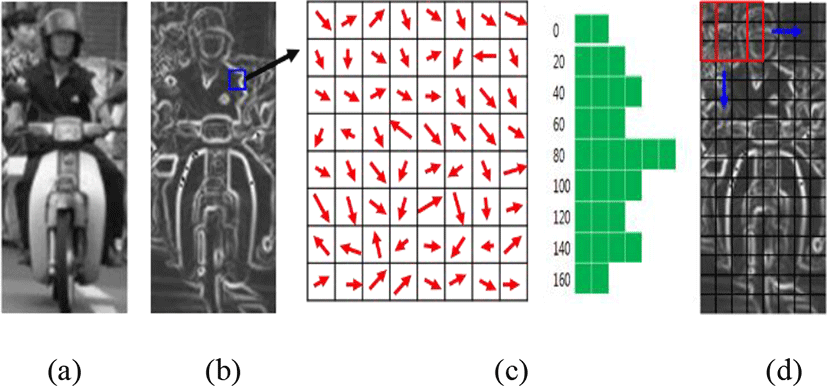Fig. 1. The example of two wheelers HOG normalization. (a) Original image (b) Calculated magnitude vector (c) cell and orientation binning expression (d) normalization by blocks for 3x3 cells
2.2 Block normalization

Directional histograms for brightness prepared in each of the cells were normalized as a block of 3x3 cells. This is performed by grouping cells in larger spatial regions called blocks. Characteristic quantities (9 dimensions) of row i, column j, Cell (i, j) is expressed as Fi,j=[F1, F1, … ,F9]. The characteristic quantities of k’th block (81 dimensions) may be expressed as:

${B}_{k}=\left[\begin{array}{l}{F}_{ij},{F}_{i+1j},{F}_{i+2j}\\ {F}_{ij+1},{F}_{ij+2},{F}_{i+1j+1}\\ {F}_{i+2j+1},{F}_{i+1j+2},{F}_{i+2j+2}\end{array}\right]$
(5)

Normalization processes are summarized in Figure 1 (d), where a movement of block is based on fact is moved to the right side and to the lower side by one cell each. The overlapping process is done to ensure the important features of each cell. The normalized characteristic vectors for each block are given by

${\Pi }_{k}=\frac{{B}_{k}}{\sqrt{{‖B‖}^{2}+{\epsilon }^{2}}}\left(\epsilon =1\right)$
(6)

And the feature vectors are saved by concatenation method. For example, the dimension number for the height and width of an input image are 128x64 pixels, the dimension number of the histogram is 9, the size of cell is 8, and the size of block is 3, then the calculated the number of HOG feature vectors with 6804 dimension is obtained.

2.2 Haar-like

The Haar-like features value, which is first proposed by Oren, et al. , is used to represent the feature of horizontal, vertical, and diagonal edge in image. The purpose of these are used as overcomplete Haar wavelets for pedestrian detection. Later, Papageorgiou & Poggio  studied the overcomplete Haar wavelets for the detection: face, car, and pedestrian. Following the idea of overcomplete Haar wavelets, Viola and Jones  proposed the Haar-like rectangle features for face detection and Lienhart and Maydt  added rotated rectangle features to the feature set, which is called extended Haar-like features. This calculate a sum of each component: the pixel gray level values sum over the black rectangle and the sum over the whole feature area, as shown in Figure 2.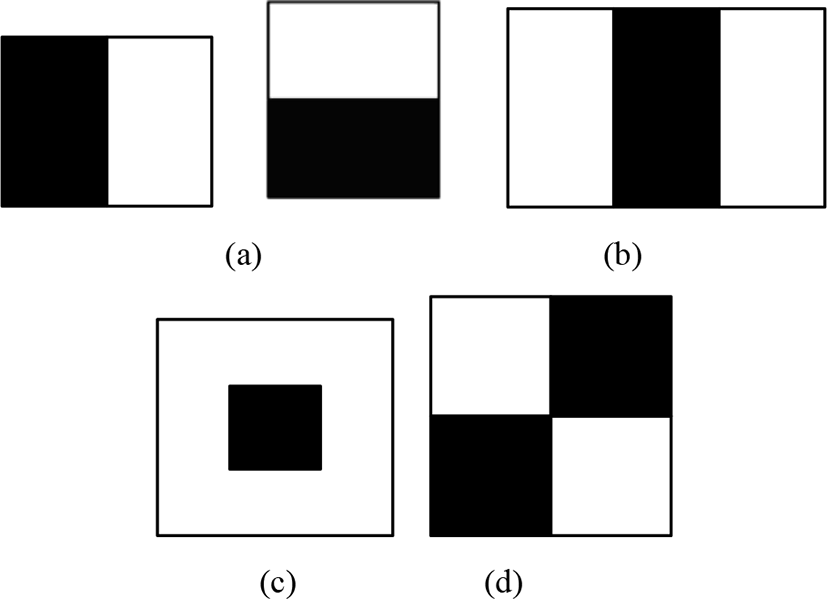Fig. 2. Subset of Haar-like features prototypes. (a) Edge feature. (b) Line feature. (c) Center-surround feature. (d) Diagonal feature.

Because in a real and robust classifier is used hundreds of features, the direct computation of pixel sum over multiple rectangles will make the detection work very slow and not suitable for real time application. Viola et al.  introduced a very effective algorithm for computing the sum quickly, so called integral image. The integral image, summed area table (SAT), is computed over the whole image I . The definition of SAT ( K(x,y) ) is following:

$K\left(x,y\right)=\sum _{{x}^{\text{'}}\le x,{y}^{\text{'}}\le y}I\left({x}^{\text{'}},{y}^{\text{'}}\right)$
(7)

The sum is done by using the rectangle corners coordinates, as described in the equation (7) and Figure 3.

$SUM\left(r\right)=K\left({x}_{4},{y}_{4}\right)-K\left({x}_{2},{y}_{2}-1\right)-K\left({x}_{3}-1,{y}_{3}\right)+K\left({x}_{1}-1,{y}_{1}-1\right)$
(8)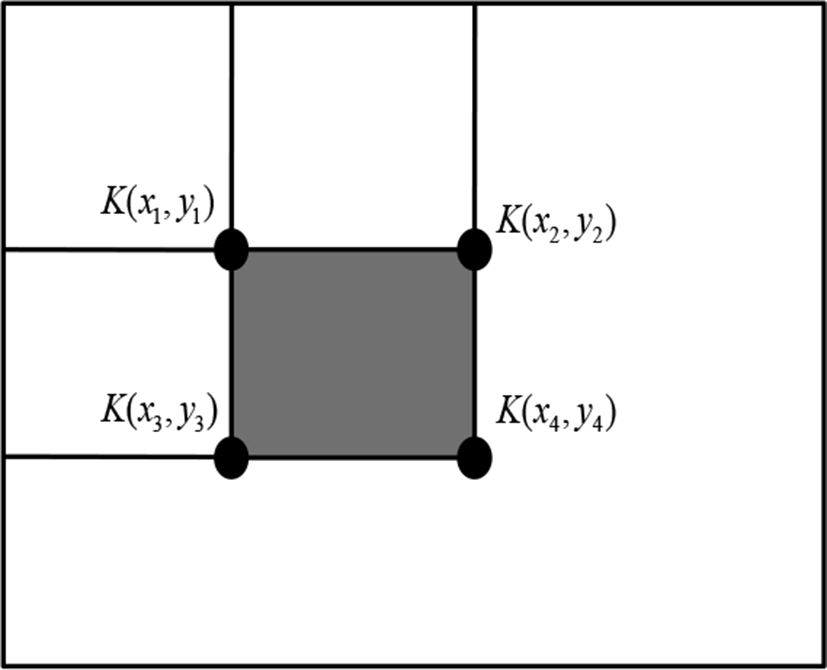Fig. 3. The calcultaion of sums.
2.3 Correlation Coefficient (CC)

A coefficient of correlation or Pearson product-moment correlation coefficient (PMCC) is a numerical measure of how much one number can be expected to be influenced by changes in another. It is expressed between -1 and 1 that measure the strength of the linear relationship between two variables. A correlation coefficient of zero means that the two numbers are not related. A non-zero correlation coefficient means that the numbers are related, but unless the coefficient is either 1 or -1 there are other influences and the relationship between the two numbers is not fixed. As previously defined, even though correlation coefficient includes the negative range, because it means that two numbers are inversely correlated, we regarded the negative value as the positive value. So this (ρ) calculator uses the following:

$\begin{array}{l}0\le |{\rho }_{cx,cy}|\le 1\hfill \\ \rho =\frac{C\left(cx,cy\right)}{{\sigma }_{cx}{\sigma }_{cy}}=\frac{C\left(cx,cy\right)}{\sqrt{{V}_{\left(cx\right)}}\sqrt{{V}_{\left(cy\right)}}}\hfill \end{array}$
(9)

where σcx,σcy is standard deviation for two cell, cx and cx , and C(cx,cy) is the covariance of two cells. In General, correlation coefficient is used to explain the information we calculate about the magnitude in the one cell by observing another magnitude in the cell. As shown in Figure 2, the cells of two wheelers area are showing different type of characteristic than other area, such as background or road area (bottom). Then we emphasize that our paper proposed an innovation methods based on the relation information of two cells

## III. CLASSIFIER

Adaboost is a simple learning algorithm that selects a small set of weak classifiers from a large number of potential features according to the weighted majority of classifiers. The training procedure of Adaboost is a greedy algorithm, which constructs an additive combination of weak classifier. Our boosting algorithm is basically the same as P. Viola’s algorithm . The boosting algorithm pseudocode for Adaboost is given in Figure 3. The algorithm takes as input a training set (x1,y1),…(xn,yn) where each xi belong to some domain X and each label yi is in some label set Y.

Given training set: (x1,y1),…(xn,yn), where xiX, yiY = {+1,−1}

1. Initialize weights ${w}_{1,i}=\frac{1}{2m},\frac{1}{2l}$ for yi = +1,−1

m: the number of negative image (pedestrian, +1)

n: the number of negative image (non-pedestrian, -1)

2. For t=1 ···T:

1. Normalize the weights,

${w}_{t,i}=\frac{{w}_{t,i}}{\sum _{j=1}^{n}{w}_{t,j}}$

so that wt,i is a probability distribution of ith training image for tth weak classification

2. For each feature, j, train a classifier hj which is restricted to using a single feature. The error is evaluated with respect to wi

${\epsilon }_{j}=\sum _{i}{w}_{j}|{h}_{j}\left({x}_{i}\right)-{y}_{i}|$

3. Choose the classifier, ht, with the lowest error εt

4. Update the weights:

${w}_{t+1,i}={w}_{t,i}{\beta }_{t}^{1-{\epsilon }_{i}}$

Where εi = −1 if example xi is classified correctly, εi = +1 otherwise, and ${\beta }_{t}^{{}_{i}}=\frac{{\epsilon }_{t}}{1-{\epsilon }_{t}}$

3. Output the final hypothesis:

$H\left(x\right)=sign\left(\sum _{t=1}^{T}{\alpha }_{t}{h}_{t}\left(x\right)\right),$

where αt = log(1/βt)

The final hypothesis H is a weighted majority vote of the T weak hypotheses where αt is the weight assigned to ht. Using two strong classifications, in this paper suggests 2nd stage cascade method. It improves the recognition rate due to the complementary role for two feature vector of quite different type.

## IV. EXPERIMENTAL RESULTS

To access the effectiveness of the proposed evolutionary method, the algorithm was applied to a practical proposed method. Our system run on a platform based on Intel Core i7(2.5 GHz) and 8GB DDR memory. For the proposed efficient two wheeler detection approach, we describe the performance of our innovative technique using correlation coefficient. We evaluate the performance of our techniques on our positive samples (two wheelers datasets; bicyclist and motorcycle driver). Then the detection performance is compared with other typical features. This system used a dataset consisting of two kinds of type according to the front view point for horizontal line: 90° and 60°. And in our study, we also experiment about mixed the 90° and 60°. Our dataset contains only front and back view with a relatively limited range of poses (60° and 90°) which are scaled to size 16x128 pixels. And the negative (non-two wheelers) samples used in our experiments were extracted randomly from general street images. All our dataset examples used in this paper shows in Figure 4. In here, “P” means positive and “N” means negative.Fig. 4. Examples of training samples for positive and negative

In Figure 5, we confirmed that the mixed two degrees (90° and 60°) showed bigger area for the receiver operating characteristic (ROC) curves than non-mixed degree. For the HOG features, we have used a cell size of 3x3 pixels with block size of 3x3 cells, descriptor 9 orientation bins of signed gradients to train Adaboost classifiers.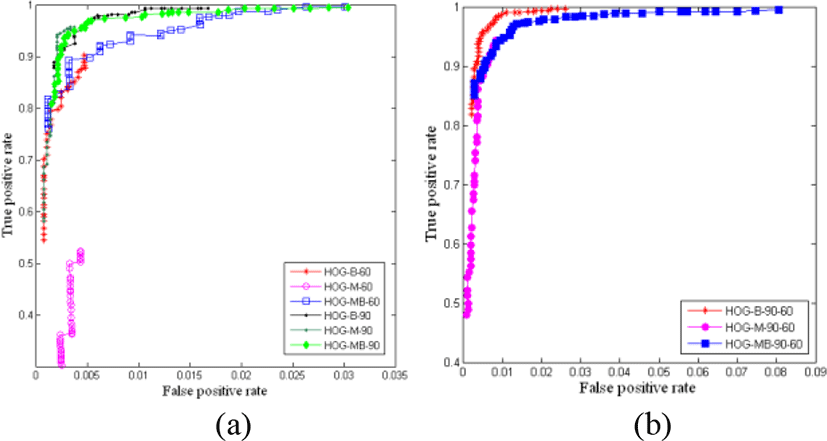Fig. 5. The ROC curves of testing samples for traditional HOG method. (a) Non-mixed degree. (b) Mixed degree.

Our preliminary experiments were tested on our two wheelers data set, and Figure 6 shows the ROC curves for the traditional HOG method. The ROC curves were generated as described in  by adjusting the threshold (θ) of the last two node of the object detector from -20 to 20. Note that in Figure 5 and 6, the true positive rate is plotted against the number of false positive detections.Fig. 6. The ROC curves of testing samples for the proposed method: HOG_CC1 and HOG_CC2. (a) HOG_CC1 non-mixed degree. (b) HOG_CC1 mixed degree. (c) HOG_CC2 non-mixed degree (d) HOG_CC2 mixed degree.

This paper tried two kinds of correlation coefficient (CC) methods: CC1 and CC2. The CC1 is calculated the CC value between each cell and special area which is showed human and two wheelers, as shown in Figure 7. In this paper, the special area was established the red region in the Figure 7 (c). The sum of CC value calculated by equation (8) is multiplied as the cell weight. This is normalized as following:

$K=\frac{1}{\sum {\rho }_{i}}$
(10)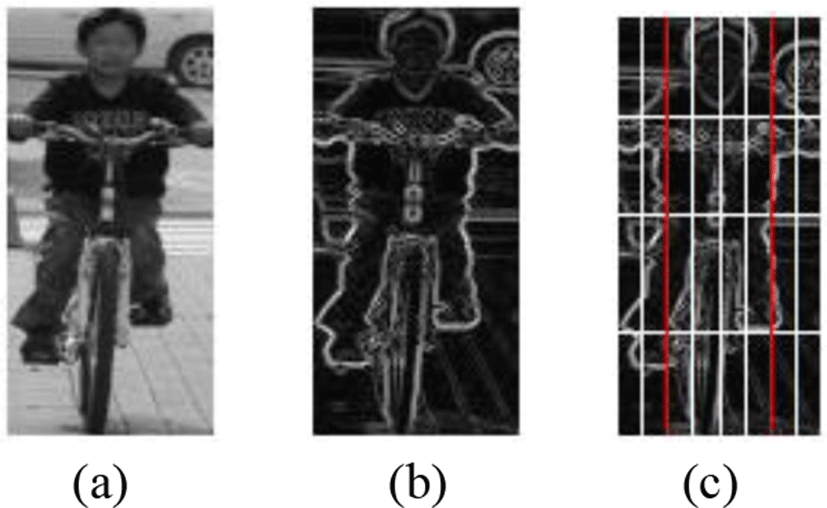Fig. 7. The example of CC1 target area for input image. (a) Original image. (b) Extracted edge image. (c) Target area for CC.

The second method, CC2 is calculated similar to CC1. But in this case, we thought that if the human and bicycle is separated to calculate the CC as the target region, it will be more enhanced performance and have remarkable features. And then, upper cell is calculated below cells and below cell are calculated upper cells, as shown Fig. 8.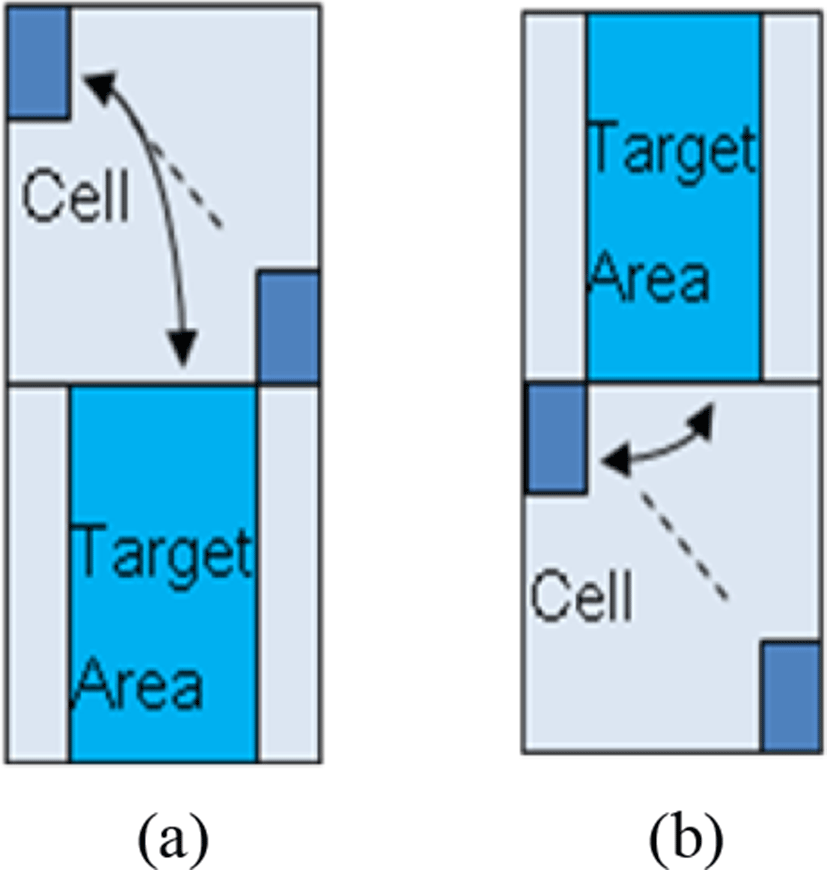Fig. 8. Proposed CC2 method to calculate the CC. (a) Upper cell to lower target area. (b) Lower cell to upper target area.

From the CC2 method, the performance reveal that the true positive rate shows near to 1 and the false positive rate under 0.04, as shown in Figure 6 ROC curves. This means that our proposed algorithm presents outstanding results than previous methods. And another excellent performance shows in table 1 which is comparison of the recognition accuracy results for the using of different feature types. In here, B means bicyclist, M means motorcycle driver, and MB means mixed bicyclist and motorcycle driver.

Table 1. The recognition results for different features (%).
Tr. rate Degree
60 90 Mixed 90 and 60
B M MB B M MB B M MB
HOG 1:1 98.64 93.24 98.27 99.17 99.41 98.82 98.99 98.76 98.04
1:2 99.18 98.33 98.34 99.20 99.44 99.00 98.97 99.12 97.94
HOG_CC1 1:1 98.61 93.99 98.38 99.09 99.19 98.87 98.68 98.79 97.85
1:2 99.00 98.90 98.64 99.15 99.38 98.78 98.59 99.15 97.83
HOG_CC2 1:1 99.94 99.90 99.94 99.87 100 99.88 99.95 99.88 99.97
1:2 99.91 99.89 99.97 99.82 99.91 99.81 99.88 99.98 99.97

In table 1, the HOG_CC1 shows similar to the traditional HOG method for each training rate. But the HOG_CC2 presents not only higher accuracy rate than the traditional HOG and HOG_CC1 method for training rate and all degree but also less calculation time than the HOG_CC1 due to using half image. Adapted equation is as follows:

$K=\sum {\rho }_{i}$
(4.2)
${C}_{j}={C}_{j}*K$
(4.2)

where i is the number of CC calculation and j means cell number. K is the sum of CC and Cj means j th cell. For the same data set, a comparison of the performance results for another different feature types are shown in Figure 9. The result of Haar-like features shows lower performance than HOG and proposed algorithms, because of concentrated left lower area in the Figure 9.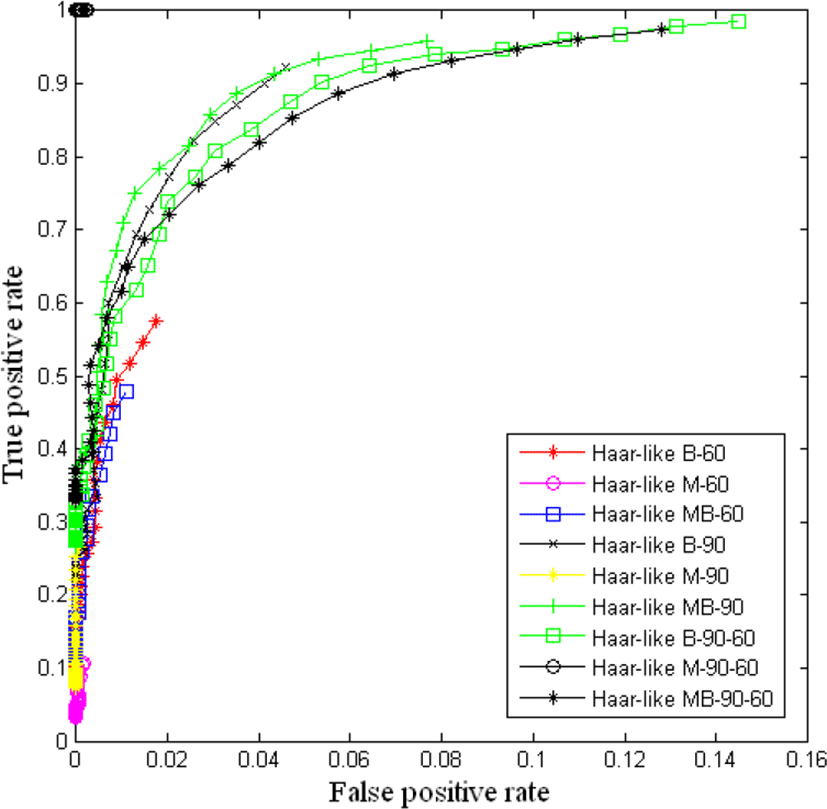Fig. 9. The result of Haar-like features.

Used each training set ratio of positive and negative is 1:1 and 1:2. For more detail, the bicyclist training images are 340 examples for 60 degrees and 845 examples for 90 degrees. And motorcycle driver training images are 96 examples for 60 degrees and 234 examples for 90 degrees. We also train the mixed of two degree and types (bicyclist and motorcycle driver). In the first experiments, the performance of the traditional HOG feature which was applied spatial regions periodicity method has shown in Figure 5, according to the data type and degree.

## V. CONCLUSION

This representation yields not only a suggested solution for weak objects (two wheelers) on the road but computationally efficient algorithm using the correlation coefficient. It has the problem that two wheelers move faster than the pedestrian on the road. So it has very valuable research to protect the human life and to avoid the accident. To solve this problem, we proposed that HOG_CC2 used a sliding window approach has outstanding detection result than previous algorithm. Adaboost classification based methods have comprised the mainstream of research to detect two wheelers and have been shown to achieve successful results in two wheelers detection. It has been experimentally demonstrated that CC2 method generated by the correlation coefficient of local features (human bicycle) leads to better classification results than other traditional methods from ROC. Furthermore, we also confirmed the recognition accuracy of HOG_CC2 has higher detection rate than HOG and HOG_CC1.

From the experimental results, we proved that the process of two wheelers detection may use smaller local features, low dimension and less computation than earlier suggested. And we consider that there are many future experiments that could be done to extend of this study (not deals with including occluded area, object change according to the weather, and others degree in this paper.

## REFERENCES

.

T. Gandhi and M. M. Trivedi, “Pedestrian Protection Systems: Issues, Survey,” and Challenges. IEEE Transaction on Intelligent Transportation Systems, Vol. 8, No.3, pp. 413-430, 2007..

S. Paisitkriangkrai, C. Shen, and J. Zhang, “Fast Pedestrian detection using a cascade of boosted covariance features,” IEEE Transaction on Circuit and System for Video Technology, Vol. 18, No.8, pp.1140-1151, 2008..

X.B. Cao, Y.W. Xu, D. Chen and H. Qiao, “Associated evolution of a support vector machine-based classifier for pedestrian detection,” Information Sciences, vol. 179, no. 8, pp.1070-1077, 2009.

H. Cho, P. E. Rybski and W. Zhang, “Vision-based bicyclist detection and tracking for intelligent vehicles,” 2010 IEEE Intelligent Vehicles Symposium, pp. 454-461, San Diego, CA., 2010.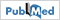.

M. Enzweiler and D.M. Gavrila, “Monocular Pedestrian Detection: Survey and Experiments,” IEEE PAMI, vol. 31, no.12, pp. 2179-2195, 2008..

D. M. Gavrila, “Sensor-based pedestrian protection,” IEEE Intelligent System, vol. 16, no. 6, pp. 77-81, 2001..

Y. Xu, X. Cao, and H. Qiao, “An Efficient Tree Classifier Ensemble-based Approach for Pedestrian Detection,” IEEE Transaction on System, Man and Cybernetics, vol. 41, no. 1, pp. 107-117, 2011..

X. Liu and K. Fujimura, “Pedestrian detection using stereo night vision,” IEEE Veh. Technol. Vol. 53, No. 6, pp. 1657-1665, 2004..

M. Bertozzi, A. Broggi, A. Lasagni and M. D. Rose, “Infrared stereo vision-based pedestrian detection,” in Proc. IEEE Intell. Veh. Symp., pp. 24-29, 2005..

H. G. Jung, Y. H. Lee, P. J. Yoon, I. Y. Hwang and J. Kim, “Sensor fusion based obstacle detection/classification for active pedestrian protection system,” in Proc. Adv. Vis. Comput., pp. 294-305, 2006..

I. P. Alonso, D. F. Llorca, M. A. Sotelo, L. M. Bergasa, P. R. de Toro, J. Nuevo, M. Ocana and A. G. Garrido, “Combination of feature extraction methods for SVM pedestrian detection. IEEE Trans. Pattern Anal. Mach. Intell., Vol. 8, No.2, 2007..

H. G. Jung, “Pedestrian Protection System: Review and Problems,” KSAE 2010 Annual Conference, pp. 1820-1826, 2010..

O. Tuzel, F. Porikli and P. Meer, “Human detection via classification on Riemannian manifolds. in Proc. IEEE conf. comp. Vis. Pattern Recognition, pp. 1-8, 2007..

L. Yu, F. Zhao and Z. An, “Locally Assembled Binary Feature with Feed-forward Cascade for pedestrian Detection in Intelligent Vehicles,” in Proc. 9th Int. Conf. on Cognitive Informatics, pp. 458-463, 2010..

L. Oliveria, P. Peixoto and U. Nunes, “A Hierarchical Fuzzy Integration of Local and Global Feature-based Classfiers to Recognize Objects in Autonomous Vehicles,” Intl. conf. on Robotic Automation, pp. 45-50, 2007..

P. F. Felzenzwalb, R. B. Girshick, D. Mcallester and D. Ramanan, “Object Detection with Discriminatively Trained Part-Based Models,” IEEE Trans. Pattern Anal. Mach. Intell., vol. 32 no.9, pp. 1627-1645, 2010..

K. Mikolajczyk, C. Schmid and A. Zisserman, “Human detection based on a probabilistic assembly of robust part detectors,” in Proc. Eur.Conf. Comput. Vis., pp. 69-81, 2004..

D. M. Gavrila and S. Munder, “Multi-cue pedestrian detection and tracking from a moving vehicle,” Int. J. Comput. Vis. Vol. 73, No. 1, pp. 41-59, 2007..

P. Sabzmeydani and G. Mori, “Detecting Pedestrians by learning shapelet features,” in Proc. CVPR, pp. 1-8, 2007..

T. Ahonen, A. Hadid and M. Pietikainen, “Face description with local binary patters: Application to face recognition”, IEEE Trans. Pattern Anal. Mach. Intell., vol. 28, no. 12, pp. 2037-2041, 2006..

N. Dalal and B. Triggs, “Histogram of Oriented Gradients for Human Detection,” IEEE Computer. Vision Pattern Recognition, pp.886-893, 2005..

C. S. Chen, C. Matsushima, Y. Yamauchi, T. Yamashita and H. Fujiyoshi, A Method for Reducing number of HOG Features based on Real Adaboost, IPSJ SIG Technical Repot, pp. 1-8, 2009..

S. Pavani, D. Delgado and A. F. Frangi, “Haar-like features with optionally weighted rectangles for rapid object detection,” Pattern Recognition, Vol. 43, No. 1, pp.160-172, 2010..

X. Y. Wang, T. X. Han and S. Yan, “An HOG-LBP human detector with partial occlusion handling,” in Proc. ICCV, pp. 32-39, 2009..

S. H. Lee, Y. H. Lee, T. S. Kim and H, D. Seo, “Pedestrian Recognition using the Cascade Method of Independent Features based on Adaboost Algorithm,” The Journal of KIIT, Vol. 8, No. 8, pp. 85-94, 2010..

D. Gerónimo, A. M. López, A. D. Sappa and T. Graf, “Survey of Pedestrian Detection for Advanced Driver Assistance Systems,” IEEE Trans. Pattern Anal. Mach. Intell., Vol. 32, No. 7, pp. 1239-1258, 2010..

S. Munder and D. M. Gavrila, “An Experimental Study on Pedestrian Classification,” IEEE Trans. Pattern Anal. Mach. Intell., Vol. 28, No. 11, pp.1-6, 2006..

Y. H. Lee, J. Y. Ko, J. H. Suk, T. M. Roh and J. C. Shim, “Pedestrian Recognition using Adaboost Algorithm based on Cascade Method by Curvature and HOG,” The Journal of KIISE, Vol. 16, No. 6, pp. 652-662, 2010..

C. Papageorgiou and T. Poggio, “A trainable system for object detection,” International Journal of Computer Vision, vol. 38, no. 1, pp.15-33, 2000..

R. Fransens, R. V. Depoortere and J. D. Prins, “Boundary based feature selection,” Technical report, 2002..

D. M. Gavrila and V. Philomin, “Real-time object detection for “smart” vehicles,” in Proc. of ICCV, Vol. 1, pp. 87-93, 1999..

P. Viloa, M. Jones and M. Snow, “Detecting pedestrians using patterns of motion and appearance. The 9th ICCV, pp. 153-161, 2003..

R. Ronfard, C. Schmid and B. Triggs, “Learning to parse pictures of people,” The 7th ECCV, pp. 700-714, 2002..

F. Porikli, “Integral histogram: a fast way to extract histograms in Cartesian spaces,” CVPR 2005, vol. 1, pp. 829-836, 2005.

.

M. Oren, C. Papageorgiou, P. Sinha, E. Osuna and T. Poggio, “Pedestrian detection using wavelet templates,” Proceedings of the IEEE Conference on Computer Vision and Pattern Recognition, pp. 193-199, 1997..

R. Lienhart and J. Maydt, “An Extended Set of Haar-like Features for Rapid Object Detection,” IEEE ICIP 2002, Vol. 1, pp. 900-903, 2002..

Y. Lee, T. Kim, S. Lee, and J. Shim, “Spatial Regions Periodicity based Detection of Two-wheelers using Histogram of Oriented Gradients,” International Journal of Multimedia and Ubiquitous Engineering, Vol.10, No.4, pp.325-336, 2015.